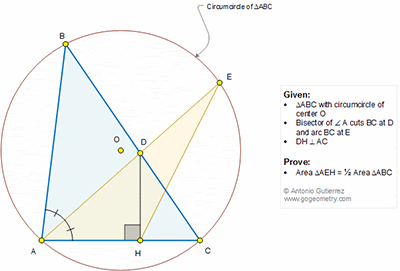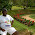## Sunday, January 8, 2017

### Geometry Problem 1305 Triangle, Circumcircle, Angle Bisector, Arc, Perpendicular, Area

Geometry Problem. Post your solution in the comment box below.
Level: Mathematics Education, High School, Honors Geometry, College.

Details: Click on the figure below.#### 6 comments:

1.Triangle ADB and ACE are similar by AA similarity.
We have AD/AB=AC/AE or AD.AE = AB.AC .

Extend DH to point D' such that DH=D'H. We get AD'=AD and <DAD'= <A.
Since H is mid-point of DD', Area of Tr. ADH = Area of Tr. AD'H, also Area of Tr. EDH = Area of Tr. ED'H . Hence Area of Tr. AEH = 1/2 Area of AD'E.

Area of Tr. AD'E = 1/2.AD'.AE. sin(A) = 1/2.AD.AE.sin(A) = 1/2.AB.AC.sin(A)=Area of Tr. ABC. We have the result, Area of Tr. AEH = 1/2.Area of Tr.ABC

2.Problem 1305
Let AF, DG are perpendicular to BK, AB respectively.Then <AGD=<AHD=<AFD so the points A,G,D,F and H are concyclic .Hence <GFB=<GFD=<GAD=<BAE=<CAE=<CBE, so
GF//BE. Similar HF//EC.If the BC intersects GE and HE at K,L respectively .
Let area triangle ABC is (ABC) then (BGK)=(FKE) and ((HLC)=(FLE).
(ABC)=(AGKLH)+(BGK)+(HLC)=(AGKLH)+(FKE)+(FLE)=(AGEH)=2.(AEH).
APOSTOLIS MANOLOUDIS KORYDALLOS PIRAEUS GREECE

3.https://goo.gl/photos/2Wk2e5vJSCUmWPte8

Draw DF ⊥ AB
Triangle AHE congruent to AFE ( case SAS) ( see sketch)
FH⊥AE=> Area(AFEH)=AE.MH…= 2.Area(AHE)..(1)
Applying Ptolemy’s theorem in quad. ABEC give us AE= (b+c).BE/BC
Note that BE/BC= AD/(2.AH) and AD.HM=AH.DH ( relation in right triangle AHD )
Replace these values to (1) we will have Area(AFEH)=1/2. DH(b+c)
Now Area(ABC)=Area(ADB)+Area(ADC)= ½.DH(b+c)
Comparing these 2 areas we will have Area(AHE)= ½.Area(ABC)

4.Let DH = h, altitude of ∆AHD from H be p, AE = q and BE = CE = d.

From Ptolemy qa = d(b+c) ….(1)
S(ADC) = ½ hb = b/(b+c).S(ABC)…(2)
S(AHE) = ½ pq….(3)
p/h = ½ a/d …(4) from similar ∆s.

(3)/(2) => S(AHE) /S(ABC). (b+c)/b = pq/(hb) = ½ qa/(bd) from (4)

Now substituting for qa/d from (1),

S(AHE)/S(ABC) . (b+c)/b = ½ (b+c)/b

Hence S(AHE) = ½ S(ABC)

Sumith Peiris
Moratuwa
Sri Lanka

5.Join BE and form the triangle ABE
m(AEB) = m(ACB) (angles in same segment) --------(1)
m(BAE) = m(EAC) = x (say) ----------(2) (given)
So triangles AEB and ACD are similar
=> AB/AE = AD/AC
=> AB.AC = AE.AD ----------(3)
Area of triangle AEH = 0.5*AE*AH*sin(x)
=> AEH = 0.5*AE*AD*cos(x)*sin(x)
=> AEH = 0.5*0.5*AE*AD*sin(2x)
=> AEH = 0.5*0.5*AB*AC*sin(2x) (from (3))
=> AEH = 0.5*(Area of ABC)

6.through AE, reflect HE,get H2E, H2 on AB;connect DH2;
area AHEH2 = 2x area AHE
connect HH2, AE perpendicular to HH2;
Through AO make diameter AK of circle O;
connect KH2, DK,HK,
also connect KB,KC,KE,
KB//DH2; KC//DH; KE//HH2;
area BDH2 =area DKH2, (KB//DH2); area DHC= area DHK, (KC//DH);
area KHH2 = area EHH2, (KE//HH2)

so area ABC =area AHKH2 =area AHEH2 =2x area AHE